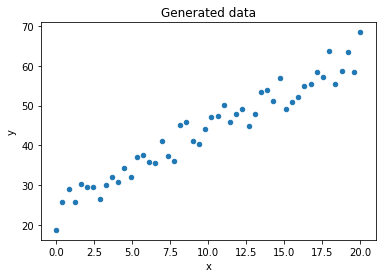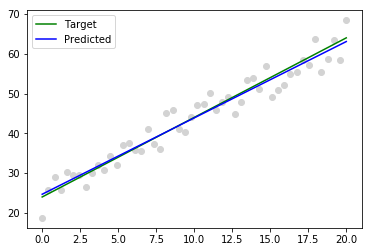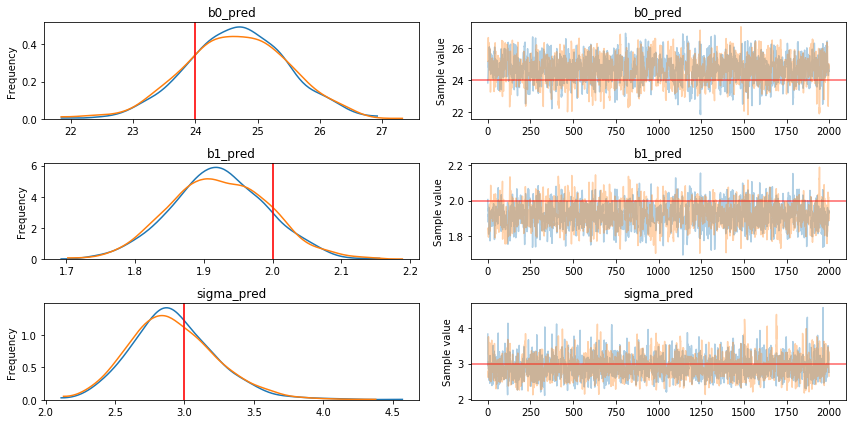## Bayesian linear regression

Find linear relationship between variables in Bayesian way (using probability to measure uncertainty)

## About linear regression model¶

Linear regression is one of the simplest and popular statistical models. Applying it we assume linear relationship between response variable(s) $y$ and explanatory variable(s) $x$. Mathematically such relationship can be written as

$$y_i = \beta_{0} + \beta_{1}x_{1i} + ... + \beta_{n}x_{ni} + \epsilon_{i}$$

where $\epsilon_{i}$ is random noise and $\beta_0, \beta_1 ... \beta_n$ - unknown coefficients (model parameters) we want to predict. It's also often assumed that $\epsilon_{i}$ is normally distributed with zero mean and unknown variance.

In case of 1 response variable $y$ and 1 explanatory variable $x$:

$$y_i = f(x_i) = \beta_{0} + \beta_{1}x_{i} + \epsilon_{i}$$

The goal of model fitting is to find unknown model parameters using the training data $(x_1,y_1),(x_2,y_2),...,(x_n, y_n)$ . After we find such parameters it's possible to run the model in direct way predicting $y_{n+1}$ with $x_{n+1}$

Non-bayesian way of finding such coefficients is optimization of a loss function (i.e. error minimization). In case of OLS it's all about minimizing the sum of the squared differences between the observed dependent variables $y_i$ and predicted ones $f(x_i)$. Such solutions give point estimates for unknown parameters. Sometimes using them is just fine. However more often we also need some measure of uncertainty. How confident we are about predicted values. Hopefully we can use bayesian inference to find unknown parameters in probabilistic way.

## Bayesian linear regression¶

We start with the same model specification

$$y_i = \beta_{0} + \beta_{1}x_{1i} + ... + \beta_{n}x_{ni} + \epsilon_{i}$$

That's equivalent to

$$y_i \sim \beta_{0} + \beta_{1}x_{1i} + ... + \beta_{n}x_{ni} + \mathcal{N}(0,\sigma^2)$$

Or

$$\mathbf{y} \sim \mathcal{N}(\mathbf{X\beta}, \sigma^2)$$

Here we treat $y$ as a random vector with the mean $\mathbf{X\beta}$ and standard deviation $\sigma$. Now it's possible to model such relationship with probabilistic programming methods.

## Example¶

Let's generate some data with Python

In :
import numpy as np
import pandas as pd

# Parameters we will try to find later with StatSim
b0 = 24
b1 = 2
sigma = 3
n = 50

# Generate X
x = np.linspace(0, 20, n)

# Set seed to get same random vector
np.random.seed(100)

# Generate Y
y = b0 + b1 * x + np.random.normal(scale=sigma, size=n)

# Show data
data = pd.DataFrame(data={'x':x,'y':y})
data.head(5)

Out:
x y
0 0.000000 18.750704
1 0.408163 25.844368
2 0.816327 29.091760
3 1.224490 25.691671
4 1.632653 30.209268

...

Draw a plot:

In :
data.plot(x='x', y='y', kind='scatter', title='Generated data');Now let's save the data and load it into StatSim

In :
data.to_csv('~/temp/linreg.csv', index=False)


## Linear regression in StatSim¶

In :
from iframer import *
iframer('https://statsim.com/app/?m=linreg-simple')


Settings:

• Chains: 3
• Samples: 5000
• Burn: 1000
• Lag: 50

## SciKitLearn¶

In :
from sklearn import datasets, linear_model

linreg = linear_model.LinearRegression()
x = x.reshape(-1,1)
y = y.reshape(-1,1)
linreg.fit(x, y)
print('b0 =', float(linreg.coef_))
print('b1 =', float(linreg.intercept_))

b0 = 1.9191940054276075
b1 = 24.685657822102073

In :
import matplotlib.pyplot as plt

y_true = b0 + b1 * x
y_predict = linreg.predict(x)
plt.scatter(x, y, color = 'lightgrey')
plt.plot(x, y_true, color = 'green', label='Target')
plt.plot(x, y_predict, color = 'blue', label='Predicted')
plt.legend();## Linear Regression model in PyMC3¶

In :
import pymc3 as pm

with pm.Model() as model:
b0_pred = pm.Uniform('b0_pred', -100, 100)
b1_pred = pm.Uniform('b1_pred', -100, 100)
sigma_pred = pm.Uniform('sigma_pred', 0.01, 10)
y_obs = pm.Normal('y_obs', mu = b0_pred + b1_pred * x, sd = sigma_pred, observed = y)
trace = pm.sample(2000, cores=2)

Auto-assigning NUTS sampler...
Initializing NUTS using jitter+adapt_diag...
Multiprocess sampling (2 chains in 2 jobs)
NUTS: [sigma_pred, b1_pred, b0_pred]
Sampling 2 chains: 100%|██████████| 5000/5000 [00:06<00:00, 730.65draws/s]
The acceptance probability does not match the target. It is 0.891548318682092, but should be close to 0.8. Try to increase the number of tuning steps.
The acceptance probability does not match the target. It is 0.9164934750561446, but should be close to 0.8. Try to increase the number of tuning steps.

In :
pm.traceplot(trace, lines={'b0_pred': b0, 'b1_pred': b1, 'sigma_pred': sigma});## Linear Regression model in TensorFlow Probability¶

In [ ]:


By Anton Zemlyansky in
Tags : #Linear regression,